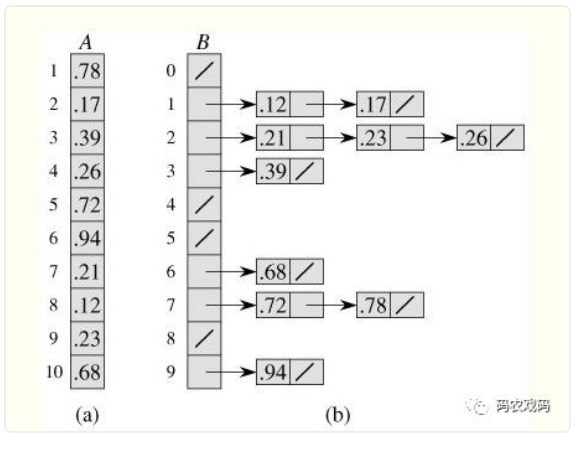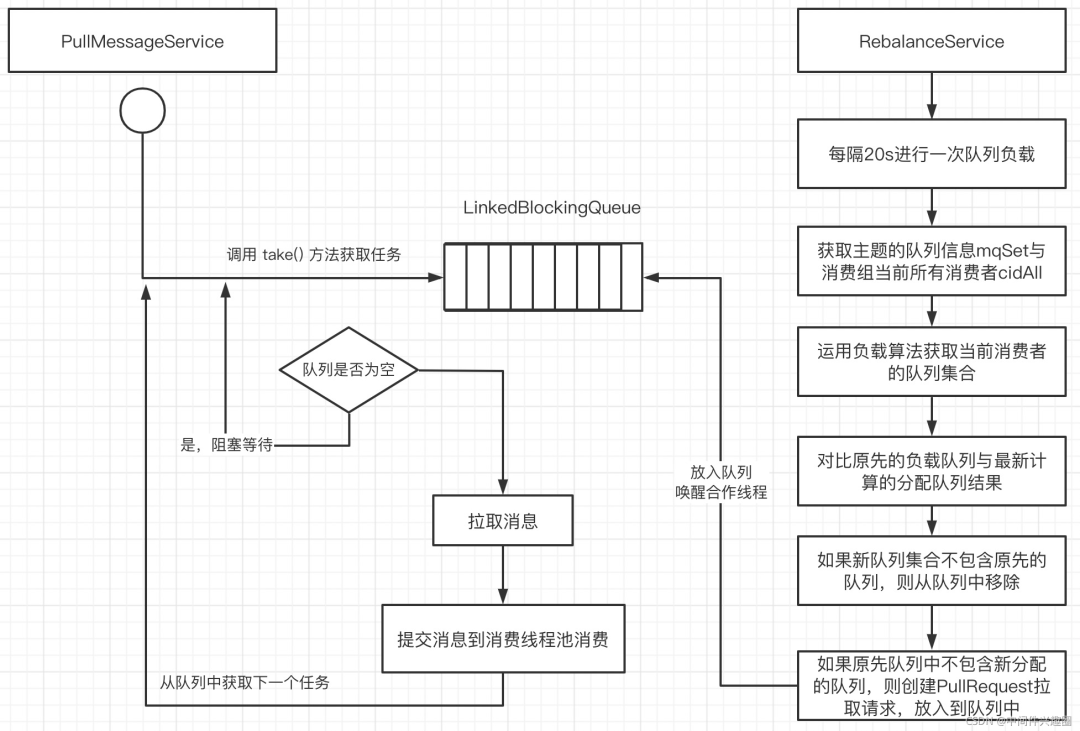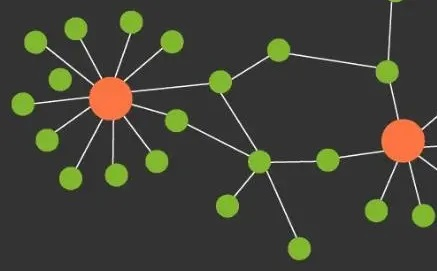# 图的遍历、拓扑排序、最短路径算法

+关注继续查看
1.DFS（深度优先搜索）

1.  访问顶点v；

2.  依次从v的未被访问的邻接点出发，对图进行深度优先遍历；直至图中和v有路径相通的顶点都被访问；

3.  若此时图中尚有顶点未被访问，则从一个未被访问的顶点出发，重新进行深度优先遍历，直到图中所有顶点均被访问过为止。

上述描述可能比较抽象，举个实例：

DFS 在访问图中某一起始顶点 v 后，由 v 出发，访问它的任一邻接顶点 w1；再从 w1 出发，访问与 w1 邻 接但还没有访问过的顶点 w2；然后再从 w2 出发，进行类似的访问，… 如此进行下去，直至到达所有的邻接顶点都被访问过的顶点 u 为止。

接着，退回一步，退到前一次刚访问过的顶点，看是否还有其它没有被访问的邻接顶点。如果有，则访问此顶点，之后再从此顶点出发，进行与前述类似的访问；如果没有，就再退回一步进行搜索。重复上述过程，直到连通图中所有顶点都被访问过为止。

//深度优先遍历：
void DFSTraverse ( Graph G )
{
visited [0 .. G.vexnum-1] = false;   // 初始化访问标志为未访问(false)
for ( v = 0; v < G.vexnum; v ++ )
if ( ! visited[v] )  DFS ( G, v );  // 从未被访问的顶点开始DFS
}

void DFS ( Graph G, int v )
{
visit ( v );  visited [v] = true;   // 访问顶点v并作标记
for ( w = FirstAdjVex(G,v); w >= 0; w = NextAdjVex(G,v,w) )
if ( ! visited[w] )  DFS ( G, w );  // 分别从每个未访问的邻接点开始DFS
}Order in which the nodes are visited (one example).

2.BFS (广度优先搜索)

算法步骤：

1.  首先将根节点放入队列中。

2.  从队列中取出第一个节点，并检验它是否为目标。

• 如果找到目标，则结束搜寻并回传结果。
• 否则将它所有尚未检验过的直接子节点加入队列中。

3.  若队列为空，表示整张图都检查过了——亦即图中没有欲搜寻的目标。结束搜寻并回传“找不到目标”。

4.  重复步骤2。

//广度优先遍历：利用队列(类似按层遍历二叉树)。
void BFSTraverse ( Graph G )
{
visited [0 .. G.vexnum-1] = false;   // 初始化访问标志为未访问(false)
InitQueue ( Q );
for ( v = 0; v < G.vexnum; v++ )
if ( ! visited[v] )
{
// 从v出发广度优先搜索
visit ( v );  visited [v] = true;
EnQueue ( Q, v );
while ( ! QueueEmpty(Q) )
{
DeQueue ( Q, u );
for ( w = FirstAdjVex(G,u); w >= 0; w = NextAdjVex(G,u,w) )
if ( ! visited[w] )
{
visit ( w );  visited [w] = true;
EnQueue ( Q, w );
}
}
}
}3.拓扑排序（topological sort）

//拓扑排序：可以测试一个有向图是否有环
void Graph::topsort( )
{
Queue<Vertex> q;
int counter = 0;
q.makeEmpty( );
for each Vertex v
if( v.indegree == 0 )
q.enqueue( v );
while( !q.isEmpty( ) )
{
Vertex v = q.dequeue( );
v.topNum = ++counter;  // Assign next number
for each Vertex w adjacent to v
if( --w.indegree == 0 )
q.enqueue( w );
}
if( counter != NUM_VERTICES )
throw CycleFoundException( );
}  

4.Dijkstra算法(Dijkstra's algorithm)

算法步骤：

1.  初始时令 S={V0},T={其余顶点}，T中顶点对应的距离值

若存在<V0,Vi>，d(V0,Vi)为<V0,Vi>弧上的权值

若不存在<V0,Vi>，d(V0,Vi)为∞

2.  从T中选取一个其距离值为最小的顶点W且不在S中，加入S

3.  对其余T中顶点的距离值进行修改：若加进W作中间顶点，从 V0 到 Vi 的距离值缩短，则修改此距离值

重复上述步骤2、3，直到S中包含所有顶点，即W=Vi 为止/*迪杰斯特拉算法：

(a) 初始化：用起点v到该顶点w的直接边(弧)初始化最短路径，否则设为∞；
(b) 从未求得最短路径的终点中选择路径长度最小的终点u：即求得v到u的最短路径；
(c) 修改最短路径：计算u的邻接点的最短路径，若(v,…,u)+(u,w)<(v,…,w)，则以(v,…,u,w)代替。
(d) 重复(b)-(c)，直到求得v到其余所有顶点的最短路径。

*/
//单源最短路径dijkstra算法：s点到其他各顶点的最短路径
void Graph::dijkstra( Vertex s )
{
for each Vertex v
{
v.dist = INFINITY;
v.known = false;
}
s.dist = 0;
for( ; ; )
{
Vertex v = smallest unknown distance vertex;
if( v == NOT_A_VERTEX )
break;
v.known = true;
for each Vertex w adjacent to v
if( !w.known )
if( v.dist + cvw < w.dist )
{
// Update w
w.dist = v.dist + cvw;
w.path = v;
}
}
}  

5.权值为1的单源最短路径

//图边的权值为1，最短路径：s点到其他各顶点的最短路径
void Graph::unweighted( Vertex s )
{
for each Vertex v
{
v.dist = INFINITY;
v.known = false;
}
s.dist = 0;
for( int currDist = 0; currDist < NUM_VERTICES; currDist++ )
for each Vertex v
if( !v.known && v.dist == currDist )
{
v.known = true;
for each Vertex w adjacent to v
if( w.dist == INFINITY )
{
w.dist = currDist + 1;
w.path = v;
}
}
}  

//图边的权值为1，最短路径：s点到其他各顶点的最短路径
void Graph::unweighted( Vertex s )
{
Queue<Vertex> q;
for each Vertex v
v.dist = INFINITY;
s.dist = 0;
q.enqueue( s );
while( !q.isEmpty( ) )
{
Vertex v = q.dequeue( );
for each Vertex w adjacent to v
if( w.dist == INFINITY )
{
w.dist = v.dist + 1;
w.path = v;
q.enqueue( w );
}
}
}  

6.有负边值的加权最短路径

//有负边值的加权最短路径算法
void Graph::weightedNegative( Vertex s )
{
Queue<Vertex> q;
for each Vertex v
v.dist = INFINITY;
s.dist = 0;
q.enqueue( s );
while( !q.isEmpty( ) )
{
Vertex v = q.dequeue( );
for each Vertex w adjacent to v
if( v.dist + cvw < w.dist )
{
// Update w
w.dist = v.dist + cvw;
w.path = v;
if( w is not already in q )
q.enqueue( w );
}
}
}  

7.计算任意两点间的最短路径

/**
* Compute all-shortest paths.
* a contains the adjacency matrix with a[ i ][ i ] presumed to be zero.
* d contains the values of the shortest path.
* Vertices are numbered starting at 0; all arrays have equal dimension.
* A negative cycle exists if d[ i ][ i ] is set to a negative value.
* Actual path can be computed using path[ ][ ].
* NOT_A_VERTEX is -1
*/
void allPairs( const matrix<int> & a, matrix<int> & d, matrix<int> & path )
{
int n = a.numrows( );
// Initialize d and path
for( int i = 0; i < n; i++ )
for( int j = 0; j < n; j++ )
{
d[ i ][ j ] = a[ i ][ j ];
path[ i ][ j ] = NOT_A_VERTEX;
}
for( int k = 0; k < n; k++ )
// Consider each vertex as an intermediate
for( int i = 0; i < n; i++ )
for( int j = 0; j < n; j++ )
if( d[ i ][ k ] + d[ k ][ j ] < d[ i ][ j ] )
{
// Update shortest path
d[ i ][ j ] = d[ i ][ k ] + d[ k ][ j ];
path[ i ][ j ] = k;
}
}  

http://www.cnblogs.com/luxiaoxun/archive/2012/08/04/2622998.html24 0《重学Java高并发》线程之间如何协作(父子线程如何优雅交互)
《重学Java高并发》线程之间如何协作(父子线程如何优雅交互)
27 083 02103 0Johnson 全源最短路径算法
1744 01608 01078 0JVM内置命令
699 0762 0
1955

0